Previous: 5. Applications Up: 5. Applications Next: 5.2 Effect of Pre-amorphization

# 5.1 Effect of Scattering Layers

Before an implantation the crystalline silicon substrate is often covered by an amorphous layer, preferably silicon dioxide, to scatter the implanted ions and to reduce thereby the channeling effect. In the following the scattering behavior of silicon dioxide (SiO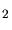), silicon nitride (Si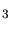N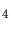) and of tungsten silicide (WSi) is analyzed, by evaluating the influence of the scattering layers on the motion of boron ions implanted with an energy of 30 keV and an energy of 90 keV.

In Fig. 5.1 to Fig. 5.3 the momentum distribution of the boron atoms leaving the scattering layer is analyzed. Even if an ion beam with ions with a single moment is implanted into a scattering layer the beam of particles leaving the scattering layer becomes divergent. This means that the distribution of the moments of the particles leaving the scattering layer is centered around the original ion beam direction, which is indicated by 0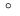in the figures Fig. 5.1 to Fig. 5.3.

If the ions were homogeneously distributed the probability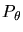for finding an ion momentum with an angle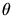to a reference direction (0) is(5.1)

The probability distributions plotted in Fig. 5.1 to Fig. 5.3 are related to such a homogeneous distribution. For instance as indicated by Fig. 5.1 (top) if the ion beam with an energy of 30 keV is scattered by a silicon dioxide layer with a thickness of 1 nm, which is approximately the thickness of a native oxide layer, the probability for ion momentums is higher compared to a homogeneous distribution for angles below 2.1while it is lower for all larger angles.

In the analysis the thickness of the scattering layer is varied from 0.5 nm to 30 nm to evaluate the scattering efficiency and the influence of the layer thickness on the scattering efficiency. The scattering efficiency of the layer is all the higher the larger the divergence of the ion beam leaving the scattering layer. The scattering efficiency of two materials can be compared by comparing the divergence of the ion beam at a fixed layer thickness.

In Fig. 5.1 the scattering efficiency of SiOis analyzed, while Fig. 5.2 and Fig. 5.3 demonstrate the scattering efficiency of SiNand WSi, respectively. Two ion energies are analyzed for each material to see if also the ion energy has an influence on the scattering efficiency.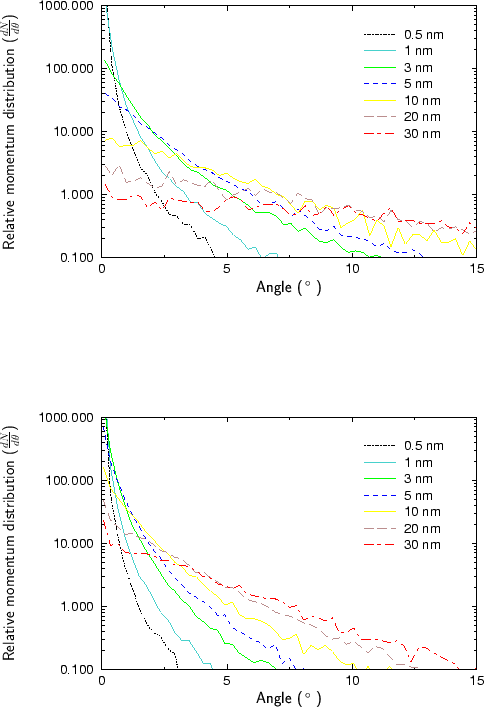According to the simulation results the divergence of the scattered ions is all the higher the thicker the scattering layer is. For instance if SiNis used as a scattering layer and ions with an energy of 90 keV are implanted the momentum distribution equals the homogeneous momentum distribution at a scattering angle of 2.2if the layer thickness is 1 nm, while it equals the homogeneous momentum distribution at a scattering angle of 6,7if the layer thickness is 20 nm (Fig. 5.2 (bottom)).

Additionally it can be said that besides the layer thickness also the ion energy has an influence on the scattering efficiency. By decreasing the energy of the ions the scattering efficiency increases. For instance, the probability for finding an ion scattered by an angle of 2,5is 1.4 times higher for an implantation energy of 30 keV than for an implantation energy of 90 keV, if a 3 nm thick WSiscattering layer is used (Fig. 5.3).

Comparing the materials SiO, SiNand WSiit can be said that WSihas the highest scattering capability while SiOhas the lowest scattering capability. While a WSilayer with a thickness of more than 10 nm is sufficient to achieve an almost homogeneous momentum distribution if the implantation energy is 30 keV, a SiOlayer of even 30 nm is not sufficient. Layer thicknesses of approximately 20 nm SiO, 4 nm SiNand 3 nm WSiare comparable in their angular scattering capabilities.

As indicated by Fig. 5.4 the stopping behavior of all scattering layers is similar to the angular scattering capability. Fig. 5.4 shows the maximum energy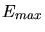of the ions leaving the scattering layer related to the implantation energy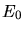. WSihas the highest stopping capability while SiOhas the lowest stopping capability of the considered materials. The stopping capability of SiNlies in between. Since the stopping power in the scattering layer is dominated by the electronic stopping (Sec. 3.3.1) as well for boron ions with an energy of 30 keV and of 90 keV, the relative energy of the scattered ions is higher for ions implanted with an energy of 90 keV (Fig. 5.4).

The reason is that the relative energy of an ion after scattering is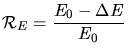(5.2)

and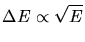(5.3)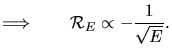(5.4)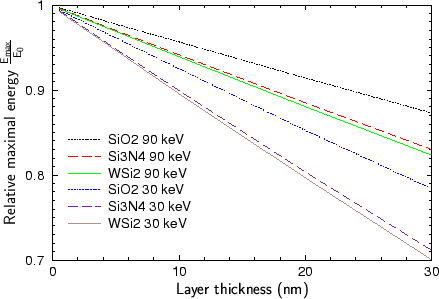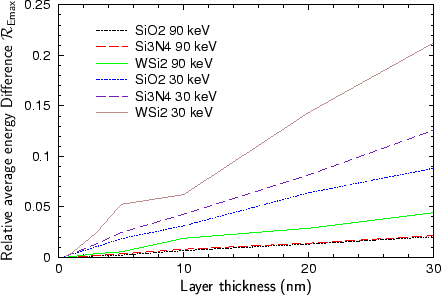According to Fig. 5.5 to Fig. 5.8 as well as the momentum distribution, the energy distribution is broadened by increasing the layer thickness or by using a material with a higher scattering capability. Fig. 5.5 shows the difference of the average energy and the maximum energy related to the maximum energy according to (5.5).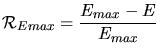(5.5)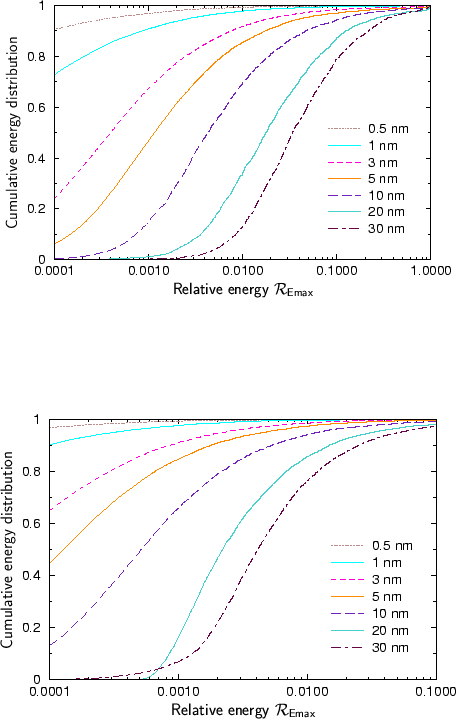In Fig. 5.6 to Fig. 5.8 the energy distributions of the boron ions leaving the scattering layer are shown. The energy distribution is presented by a cumulative sum as a function of the relative energy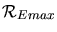compared to the maximal energyof an ion leaving the scattering layer. Using Fig. 5.6 to Fig. 5.8 the scattering capabilities of the various materials (SiO, SiNand WSi) can be analyzed in detail. As for the evaluation of the momentum distribution two implantation energies are considered for analyzing the energy distributions for each scattering material type.

The influence of the layer thickness and of the implantation energy on the energy distributions of the boron ions leaving the scattering layer is equivalent to the influence on the momentum distribution. As well by increasing the thickness of the scattering layer as by reducing the implantation energy, the energy distribution is broadened. Additionally the energy distribution is broadened by using a material with a higher scattering capability. For instance if the boron ions are implanted with an energy of 90 keV through a scattering layer with a thickness of 30 nm approximately 77% of all ions are within an energy interval of 1% around the maximal energy if the scattering layer material is SiO, while just 62% and 41% of the ions are within this interval if the scattering layer material is SiNor WSirespectively.

The influence of the scattering layers on the channeling behavior of the ions in the crystalline silicon substrate and thereby on the distribution of the implanted atoms is shown in Fig. 5.9 to Fig. 5.14 for implantations with energies of 30 keV and 90 keV and a dose of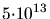cm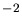. The implantations are performed on the one hand side with an ion beam tilted by 7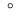and on the other hand side with an ion beam aligned with the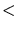100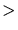crystal direction.

In case of an implantation along the100channeling direction as shown for instance in the bottom figure of Fig. 5.9 the depth of the implanted profile is successively reduced by increasing the thickness of the scattering layer. Tab. 5.1 summarizes the depth of the concentration level of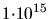cm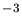after an implantation through a scattering layer with a thickness of 30 nm. Various scattering materials and two implantation energies are used. A depth of 0 nm in Fig. 5.9 to Fig. 5.14 and in Tab. 5.1 corresponds to the surface of the silicon substrate. The values of the depth in Tab. 5.1 are extracted from the top figures in Fig. 5.9 to Fig. 5.14.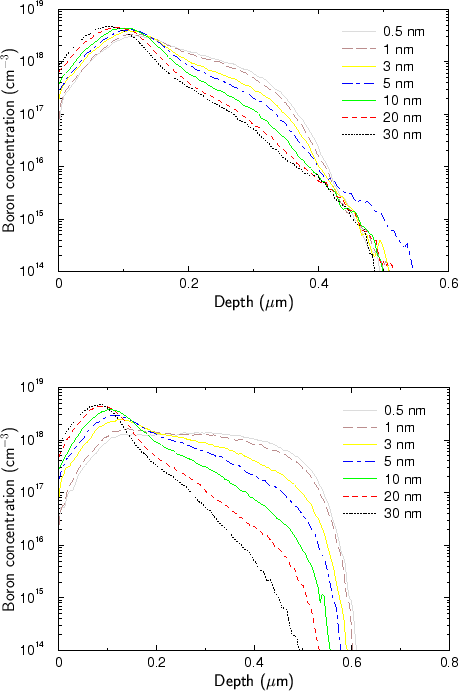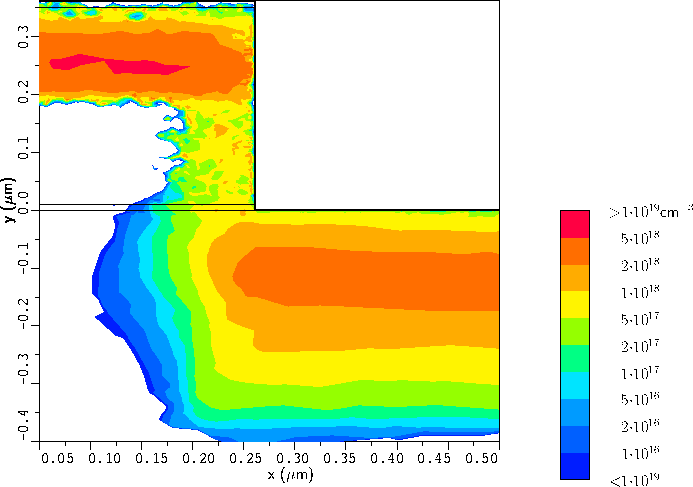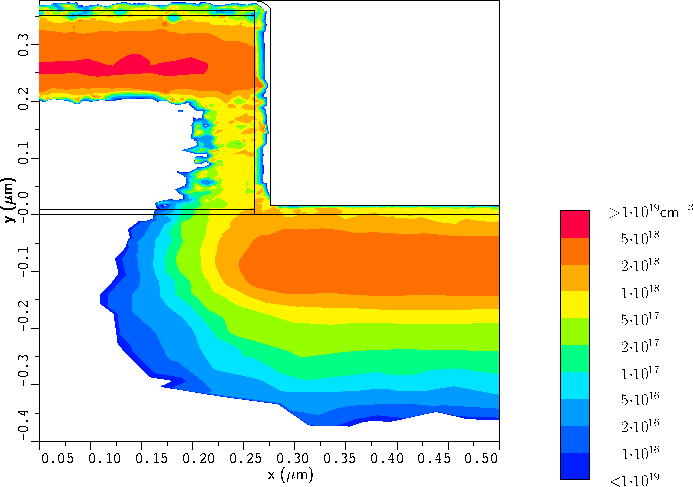If the ion beam is tilted by 7the ions first penetrate deeper into the target if the thickness of the scattering layer is increased, because the probability that an ion is scattered into a channel increases as shown in Fig. 5.1 to Fig. 5.3. At a certain scattering layer thickness the penetration depth reaches a maximum until it decreases again. If thick scattering layers are used the impurity distributions become independent on the tilt of the ion beam. To achieve that a thickness of 30 nm, which is the largest layer thickness in the analysis presented here is sufficient as well for an implantation with an energy of 30 keV and of 90 keV and for all scattering layer materials analyzed. Since the effects observed do not depend on the material type of the scattering layer a presentation just of Fig. 5.9 would be sufficient, but Fig. 5.10, Fig. 5.11, Fig. 5.12, Fig. 5.13 and Fig. 5.14 are added for the sake of completeness. In this series of figures first the tilt angle then the material type and finally the implantation energy is varied.

In order to demonstrate that such an analysis is not restricted to one-dimensional problems, the effect of a silicon dioxide scattering layer on the doping profile in the vicinity of a gate corner of a MOS transistor is shown in Fig. 5.15. The scattering layer covers the whole surface and has a thickness of 1 nm in the top figure and of 16 nm in the bottom figure.

Boron ions with an energy of 30 keV and a dose ofcmwere implanted into this structure. The ion beam was tilted by 10to move the boron atoms slightly under the gate.

By using a thicker scattering oxide the shape of the doping profile below the gate corner changes from a rectangular shaped structure to a circular shaped structure. Additionally a shallower doping profile is generated by implanting through a scattering oxide with a thickness of 16 nm. The iso-concentration line of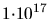cmhas a depth of approximately 280 nm and its maximal distance from the gate corner is 90 nm. In case of an implantation through a scattering layer with a thickness of just 1 nm the depth of the iso-concentration line ofcmis 355 nm and the distance from the corner is 100 nm. Therefore the aspect ratios of the doping profiles are 3.1 and 3.55 respectively.

A. Hoessiger: Simulation of Ion Implantation for ULSI Technology